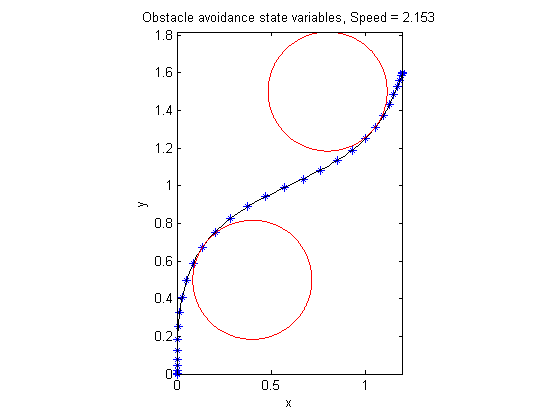74  Obstacle Avoidance

OPTRAGEN 1.0 A MATLAB Toolbox for Optimal Trajectory Generation, Raktim Bhattacharya, Texas A&M University (Note: There is typographical error in the OPTRAGEN documentation. The objective involves second derivatives of x and y.)

A robot with obstacles in 2D space. Travel from point A to B using minimum energy.

74.1  Problem Formulation

Find theta(t) and V over t in [0; 1 ] to minimize

 1 0
( (
 d2x dt2
)2 + (
 d2y dt2
)2 ) dt

subject to:

 dx dt
= V*cos(theta
 dy dt
= V*sin(theta
 [x0  y0] = [0  0]
 [x1  y1] = [1.2  1.6]
 (x−0.4)2 + (y−0.5)2 >= 0.1
 (x−0.8)2 + (y−1.5)2 >= 0.1

Where V is a constant scalar speed.

Reference: 

74.2  Solve the problem, using a successively larger number collocation points

for n=[4 15 30]
% Create a new phase and states, using n collocation points
p = tomPhase('p', t, 0, t_f, n);
setPhase(p);
tomStates x y vx vy
tomControls theta

% Interpolate an initial guess for the n collocation points
x0 = {V == speed
icollocate({x == xopt; y == yopt; vx == vxopt; vy == vyopt})
collocate(theta == thetaopt)};

% Box constraints
cbox = {0 <= V <= 100 };

% Boundary constraints
cbnd = {initial({x == 0; y == 0})
final({x == 1.2; y == 1.6})};

% ODEs and path constraints
ode = collocate({
dot(x) == vx == V*cos(theta)
dot(y) == vy == V*sin(theta)
});

% A 30th order polynomial is more than sufficient to give good
% accuracy. However, that means that mcollocate would only check
% about 60 points. In order to make sure we don't hit an obstacle,
% we check 300 evenly spaced points instead, using atPoints.
obstacles = atPoints(linspace(0,t_f,300), {
(x-0.4)^2 + (y-0.5)^2 >= 0.1
(x-0.8)^2 + (y-1.5)^2 >= 0.1});

% Objective: minimum energy.
objective = integrate(dot(vx)^2+dot(vy)^2);

74.3  Solve the problem

options = struct;
options.name = 'Obstacle avoidance';
constr = {cbox, cbnd, ode, obstacles};
solution = ezsolve(objective, constr, x0, options);

% Optimal x, y, and speed, to use as starting guess in the next iteration
xopt = subs(x, solution);
yopt = subs(y, solution);
vxopt = subs(vx, solution);
vyopt = subs(vy, solution);
thetaopt = subs(theta, solution);
speed = subs(V,solution);
Problem type appears to be: qpcon
Starting numeric solver
===== * * * =================================================================== * * *
TOMLAB - Tomlab Optimization Inc. Development license  999001. Valid to 2011-02-05
=====================================================================================
Problem: ---  1: Obstacle avoidance             f_k      29.812856165009947000
sum(|constr|)      0.000000001309307815
f(x_k) + sum(|constr|)     29.812856166319254000
f(x_0)      0.000000000000062528

Solver: snopt.  EXIT=0.  INFORM=1.
SNOPT 7.2-5 NLP code
Optimality conditions satisfied

FuncEv    1 ConstrEv   22 ConJacEv   22 Iter   20 MinorIter 2732
CPU time: 0.609375 sec. Elapsed time: 0.688000 sec.
Problem type appears to be: qpcon
Starting numeric solver
===== * * * =================================================================== * * *
TOMLAB - Tomlab Optimization Inc. Development license  999001. Valid to 2011-02-05
=====================================================================================
Problem: ---  1: Obstacle avoidance             f_k      22.128728366250083000
sum(|constr|)      0.000000000006744707
f(x_k) + sum(|constr|)     22.128728366256826000
f(x_0)     29.812856165010601000

Solver: snopt.  EXIT=0.  INFORM=1.
SNOPT 7.2-5 NLP code
Optimality conditions satisfied

FuncEv    1 ConstrEv  151 ConJacEv  151 Iter  136 MinorIter  488
CPU time: 2.437500 sec. Elapsed time: 2.703000 sec.
Problem type appears to be: qpcon
Starting numeric solver
===== * * * =================================================================== * * *
TOMLAB - Tomlab Optimization Inc. Development license  999001. Valid to 2011-02-05
=====================================================================================
Problem: ---  1: Obstacle avoidance             f_k      22.091923280888466000
sum(|constr|)      0.000000000011942997
f(x_k) + sum(|constr|)     22.091923280900410000
f(x_0)     22.128728366249423000

Solver: snopt.  EXIT=0.  INFORM=1.
SNOPT 7.2-5 NLP code
Optimality conditions satisfied

FuncEv    1 ConstrEv  289 ConJacEv  289 Iter  261 MinorIter  697
CPU time: 9.437500 sec. Elapsed time: 9.922000 sec.
end

74.4  Plot result

figure(1)
th = linspace(0,2*pi,500);
x1 = sqrt(0.1)*cos(th)+0.4;
y1 = sqrt(0.1)*sin(th)+0.5;
x2 = sqrt(0.1)*cos(th)+0.8;
y2 = sqrt(0.1)*sin(th)+1.5;
ezplot(x,y);
hold on
plot(x1,y1,'r',x2,y2,'r');
hold off
xlabel('x');
ylabel('y');
title(sprintf('Obstacle avoidance state variables, Speed = %2.4g',speed));
axis image